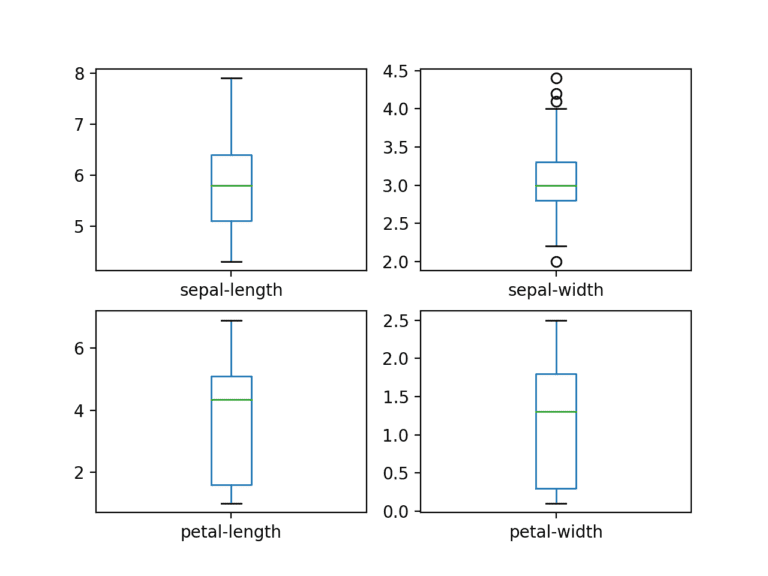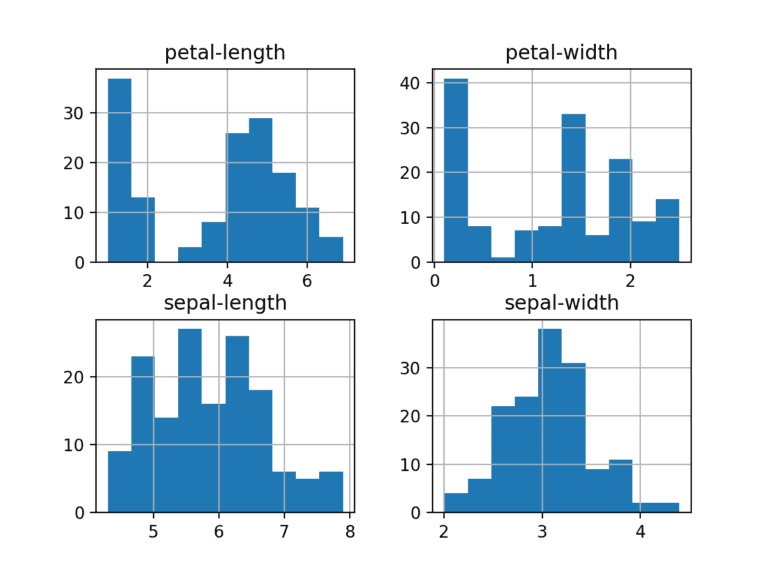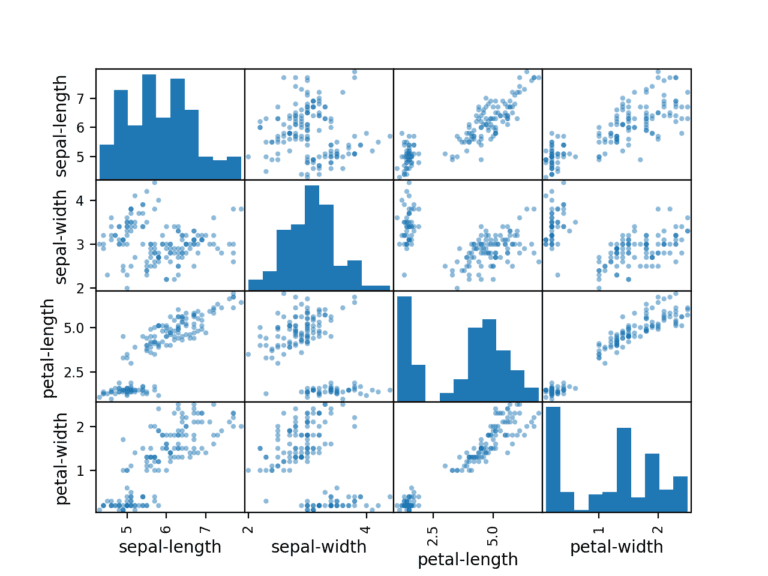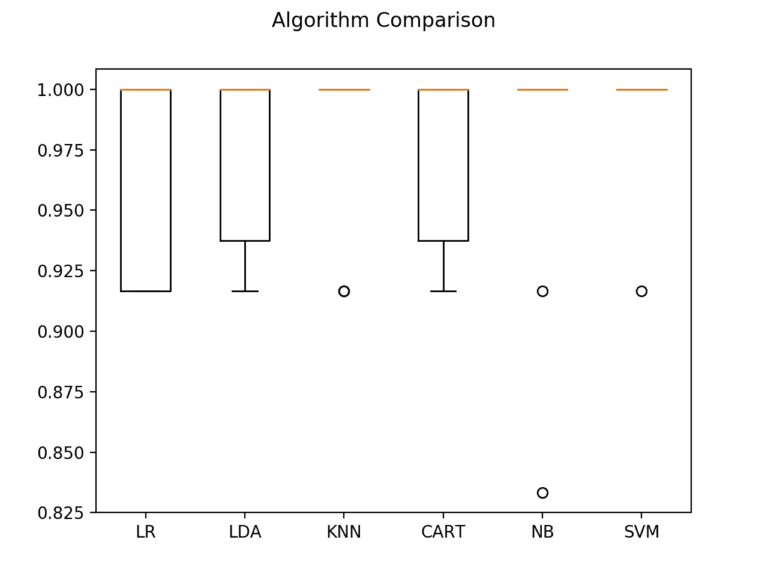# 30 minutes to machine learning

• Difficulty Level : Hard
• Last Updated : 18 Oct, 2021

Get the Python and SciPy platform installed on your system if it is not already. One can easily follow the installation guide for it.

### 1.1 Install SciPy Libraries:

Working on Python version 2.7 or 3.5+.
There are 5 key libraries that you will need to install. Below is a list of the Python SciPy libraries required for this tutorial:

• scipy
• numpy
• matplotlib
• pandas
• sklearn

### 1.2 Start Python and Check Versions:

It is a good idea to make sure your Python environment was installed successfully and is working as expected.
The script below will help to test out the environment. It imports each library required in this tutorial and prints the version.
Type or copy and paste the following script:

## Python3

 `# Check the versions of libraries` `# Python version``import` `sys``print``(``'Python: {}'``.``format``(sys.version))``# scipy``import` `scipy``print``(``'scipy: {}'``.``format``(scipy.__version__))``# numpy``import` `numpy``print``(``'numpy: {}'``.``format``(numpy.__version__))``# matplotlib``import` `matplotlib``print``(``'matplotlib: {}'``.``format``(matplotlib.__version__))``# pandas``import` `pandas``print``(``'pandas: {}'``.``format``(pandas.__version__))``# scikit-learn``import` `sklearn``print``(``'sklearn: {}'``.``format``(sklearn.__version__))`

If an error arises, stop. Now is the time to fix it.

## 2. Load The Data:

Dataset – Iris data

It is famous data used as the “hello world” dataset in machine learning and statistics by pretty much everyone.
The dataset contains 150 observations of iris flowers. There are four columns of measurements of the flowers in centimeters. The fifth column is the species of the flower observed. All observed flowers belong to one of three species.

### 2.1 Import libraries:

First, let’s import all of the modules, functions, and objects to be used.

## Python3

 `# Load libraries` `import` `pandas``from` `pandas.plotting ``import` `scatter_matrix``import` `matplotlib.pyplot as plt``from` `sklearn ``import` `model_selection``from` `sklearn.metrics ``import` `classification_report``from` `sklearn.metrics ``import` `confusion_matrix``from` `sklearn.metrics ``import` `accuracy_score``from` `sklearn.linear_model ``import` `LogisticRegression``from` `sklearn.tree ``import` `DecisionTreeClassifier``from` `sklearn.neighbors ``import` `KNeighborsClassifier``from` `sklearn.discriminant_analysis ``import` `LinearDiscriminantAnalysis``from` `sklearn.naive_bayes ``import` `GaussianNB``from` `sklearn.svm ``import` `SVC`

A working SciPy environment is required before continuing.

### 2.2 Load Dataset

Data can directly be loaded into the UCI Machine Learning repository.
Using pandas to load the data and exploring descriptive statistics and data visualization.

Note: The names of each column are specified when loading the data. This will help later at the time of exploring the data.

## Python3

 `url ``=``"https://raw.githubusercontent.com / jbrownlee / Datasets / master / iris.csv"``names ``=` `[``'sepal-length'``, ``'sepal-width'``, ``'petal-length'``,``         ``'petal-width'``, ``'class'``]``dataset ``=` `pandas.read_csv(url, names ``=` `names)`

If you do have network problems, you can download the iris.csv file into your working directory and load it using the same method, changing the URL to the local file name.

## 3. Summarize the Dataset:

Now it is time to take a look at the data.
Steps to look at the data in a few different ways:

• Dimensions of the dataset.
• Peek at the data itself.
• Statistical summary of all attributes.
• Breakdown of the data by the class variable.

## Python3

 `# shape``print``(dataset.shape)`

`(150, 5)`

## Python3

 `# head``print``(dataset.head(``20``))`

```    sepal-length  sepal-width  petal-length  petal-width        class
0            5.1          3.5           1.4          0.2  Iris-setosa
1            4.9          3.0           1.4          0.2  Iris-setosa
2            4.7          3.2           1.3          0.2  Iris-setosa
3            4.6          3.1           1.5          0.2  Iris-setosa
4            5.0          3.6           1.4          0.2  Iris-setosa
5            5.4          3.9           1.7          0.4  Iris-setosa
6            4.6          3.4           1.4          0.3  Iris-setosa
7            5.0          3.4           1.5          0.2  Iris-setosa
8            4.4          2.9           1.4          0.2  Iris-setosa
9            4.9          3.1           1.5          0.1  Iris-setosa
10           5.4          3.7           1.5          0.2  Iris-setosa
11           4.8          3.4           1.6          0.2  Iris-setosa
12           4.8          3.0           1.4          0.1  Iris-setosa
13           4.3          3.0           1.1          0.1  Iris-setosa
14           5.8          4.0           1.2          0.2  Iris-setosa
15           5.7          4.4           1.5          0.4  Iris-setosa
16           5.4          3.9           1.3          0.4  Iris-setosa
17           5.1          3.5           1.4          0.3  Iris-setosa
18           5.7          3.8           1.7          0.3  Iris-setosa
19           5.1          3.8           1.5          0.3  Iris-setosa```

### 3.3 Statistical Summary

This includes the count, mean, the min and max values as well as some percentiles.

## Python3

 `# descriptions``print``(dataset.describe())`

It is clearly visible that all of the numerical values have the same scale (centimeters) and similar ranges between 0 and 8 centimeters.

```       sepal-length  sepal-width  petal-length  petal-width
count    150.000000   150.000000    150.000000   150.000000
mean       5.843333     3.054000      3.758667     1.198667
std        0.828066     0.433594      1.764420     0.763161
min        4.300000     2.000000      1.000000     0.100000
25%        5.100000     2.800000      1.600000     0.300000
50%        5.800000     3.000000      4.350000     1.300000
75%        6.400000     3.300000      5.100000     1.800000
max        7.900000     4.400000      6.900000     2.500000```

## Python3

 `# class distribution``print``(dataset.groupby(``'class'``).size())`

```class
Iris-setosa        50
Iris-versicolor    50
Iris-virginica     50```

## 4. Data Visualization

Using two types of plots:

1. Univariate plots to better understand each attribute.
2. Multivariate plots to better understand the relationships between attributes.

### 4.1 Univariate Plots

Univariate plots – plots of each individual variable.
Given that the input variables are numeric, we can create box and whisker plots of each.

## Python3

 `# box and whisker plots``dataset.plot(kind ``=``'box'``, subplots ``=` `True``,``             ``layout ``=``(``2``, ``2``), sharex ``=` `False``, sharey ``=` `False``)``plt.show()`Creating a histogram of each input variable to get an idea of the distribution.

## Python3

 `# histograms``dataset.hist()``plt.show()`

It looks like perhaps two of the input variables have a Gaussian distribution. This is useful to note as we can use algorithms that can exploit this assumption.### 4.2 Multivariate Plots

Interactions between the variables.
First, let’s look at scatterplots of all pairs of attributes. This can be helpful to spot structured relationships between input variables.

## Python3

 `# scatter plot matrix``scatter_matrix(dataset)``plt.show()`

Note the diagonal grouping of some pairs of attributes. This suggests a high correlation and a predictable relationship.## 5. Evaluate Some Algorithms

Creating some models of the data and estimate their accuracy on unseen data.

1. Separate out a validation dataset.
2. Set up the test harness to use 10-fold cross-validation.
3. Build 5 different models to predict species from flower measurements
4. Select the best model.

### 5.1 Create a Validation Dataset

Using statistical methods to estimate the accuracy of the models that we create on unseen data. A concrete estimate of the accuracy of the best model on unseen data is taken by evaluating it on actual unseen data.
Some data is used as testing data that the algorithms will not get to see and this data is used to get a second and independent idea of how accurate the best model might actually be.
Testing data is split into two, 80% of which we will use to train our models and 20% that we will hold back as a validation dataset.

## Python3

 `# Split-out validation dataset``array ``=` `dataset.values``X ``=` `array[:, ``0``:``4``]``Y ``=` `array[:, ``4``]``validation_size ``=` `0.20``seed ``=` `7``X_train, X_validation, Y_train, Y_validation ``=` `model_selection.train_test_split(``        ``X, Y, test_size ``=` `validation_size, random_state ``=` `seed)`

X_train and Y_train are the training data for preparing models and X_validation and Y_validation sets can be used later.

### 5.2 Test Harness

Using 10-fold cross-validation to estimate accuracy. This will split our dataset into 10 parts, train on 9 and test on 1 and repeat for all combinations of train-test splits.

## Python3

 `# Test options and evaluation metric``seed ``=` `7``scoring ``=` `'accuracy'`

‘Accuracy’ metric is used to evaluate models. It is the ratio of the number of correctly predicted instances divided by the total number of instances in the dataset multiplied by 100 to give a percentage (e.g. 95% accurate).

### 5.3 Build Models

Which algorithms would be good on this problem or what configurations to use, is not known. So, an idea is taken from the plots that some of the classes are partially linearly separable in some dimensions.
Evaluating 6 different algorithms:

• Logistic Regression (LR)
• Linear Discriminant Analysis (LDA)
• K-Nearest Neighbors (KNN).
• Classification and Regression Trees (CART).
• Gaussian Naive Bayes (NB).
• Support Vector Machines (SVM).

The algorithms chosen are a mixture of linear (LR and LDA) and nonlinear (KNN, CART, NB, and SVM) algorithms. Random number seeds are reset before each run to ensure that the evaluation of each algorithm is performed using exactly the same data splits. It ensures the results are directly comparable.
Building and evaluating the models:

## Python3

 `# Spot Check Algorithms``models ``=` `[]``models.append((``'LR'``, LogisticRegression(solver ``=``'liblinear'``, multi_class ``=``'ovr'``)))``models.append((``'LDA'``, LinearDiscriminantAnalysis()))``models.append((``'KNN'``, KNeighborsClassifier()))``models.append((``'CART'``, DecisionTreeClassifier()))``models.append((``'NB'``, GaussianNB()))``models.append((``'SVM'``, SVC(gamma ``=``'auto'``)))` `# evaluate each model in turn``results ``=` `[]``names ``=` `[]` `for` `name, model ``in` `models:``    ``kfold ``=` `model_selection.KFold(n_splits ``=` `10``, random_state ``=` `seed)``    ``cv_results ``=` `model_selection.cross_val_score(``            ``model, X_train, Y_train, cv ``=` `kfold, scoring ``=` `scoring)``    ``results.append(cv_results)``    ``names.append(name)``    ``msg ``=` `"% s: % f (% f)"` `%` `(name, cv_results.mean(), cv_results.std())``    ``print``(msg)`

### 5.4 Select Best Model

Comparing the models to each other and select the most accurate. Running the example above to get the following raw results:

```LR: 0.966667 (0.040825)
LDA: 0.975000 (0.038188)
KNN: 0.983333 (0.033333)
CART: 0.975000 (0.038188)
NB: 0.975000 (0.053359)
SVM: 0.991667 (0.025000)```

Support Vector Machines (SVM) has the largest estimated accuracy score.
The plot of the model evaluation results is created and compares the spread and the mean accuracy of each model. There is a population of accuracy measures for each algorithm because each algorithm was evaluated 10 times (10 fold cross-validation).

## Python3

 `# Compare Algorithms``fig ``=` `plt.figure()``fig.suptitle(``'Algorithm Comparison'``)``ax ``=` `fig.add_subplot(``111``)``plt.boxplot(results)``ax.set_xticklabels(names)``plt.show()`

Box and whisker plots are squashed at the top of the range, with many samples achieving 100% accuracy.### 6. Make Predictions

The KNN algorithm is very simple and was an accurate model based on our tests.
Running the KNN model directly on the validation set and summarizing the results as a final accuracy score, a confusion matrix, and a classification report.

## Python3

 `# Make predictions on validation dataset``knn ``=` `KNeighborsClassifier()``knn.fit(X_train, Y_train)``predictions ``=` `knn.predict(X_validation)``print``(accuracy_score(Y_validation, predictions))``print``(confusion_matrix(Y_validation, predictions))``print``(classification_report(Y_validation, predictions))`

Accuracy is 0.9 or 90%. The confusion matrix provides an indication of the three errors made. Finally, the classification report provides a breakdown of each class by precision, recall, f1-score, and support showing excellent results (granted the validation dataset was small).

```0.9
[[ 7  0  0]
[ 0 11  1]
[ 0  2  9]]
precision    recall  f1-score   support

Iris-setosa       1.00      1.00      1.00         7
Iris-versicolor       0.85      0.92      0.88        12
Iris-virginica       0.90      0.82      0.86        11

micro avg       0.90      0.90      0.90        30
macro avg       0.92      0.91      0.91        30
weighted avg       0.90      0.90      0.90        30```

My Personal Notes arrow_drop_up